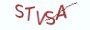Java基础教程

# 9.2.1 字符串常用操作方法

字符串类型是一种抽象数据类型，抽象数据类型定义了数据类型的操作方法，在本节的内容中，着重介绍Java字符串类的常用操作方法。

# 9.2.2 获取字符串长度

(1) String.length()

```public class HelloJava{
public  static void main(String[] args) {
String content = "好好学Java，天天向上";
// 字符串对象的length方法返回值为整型，表示字符串的字符数
int length = content.length();
System.out.println(length);
}
}```

# 9.2.3 字符串的索引访问

(1) String.charAt(int index)

```public class HelloJava{
public  static void main(String[] args) {
String content = "好好学Java,天天向上。问题来了，你为什么要学Java?";
int start = 0, end = content.length()-1;
// 访问主串中的第一个字符
System.out.println(content.charAt(start));
// 访问主串中的最后一个字符
System.out.println(content.charAt(end));
}```

# 9.2.4 字符串的大小写转换

(1) String.toLowerCase()

```public class HelloJava{
public  static void main(String[] args) {
String content = "你为什么要学Java";

String content_lower = content.toLowerCase();
System.out.println(content_lower);
}
}```

(2) String.toUpperCase()

```public class HelloJava{
public  static void main(String[] args) {
String content = "你为什么要学Java";

String content_upper = content.toUpperCase();
System.out.println(content_upper);
}
}```

# 9.2.5 字符串的子串查找

(1) String.indexOf(String str)

```public class HelloJava{
public  static void main(String[] args) {
String content = "你为什么要学Java";
// indexOf方法的返回值为整型，表示子串在主串中的起始位置
int pos = content.indexOf("Java");
System.out.println(pos);
}
}```

(2) String.indexOf(String str，int fromIndex)

```public class HelloJava{
public  static void main(String[] args) {
String content = "好好学Java,天天向上。问题来了，你为什么要学Java?";
int count = 0;
String sub = "Java";
int index = content.indexOf(sub);
while ( index != -1 ){
// 如果index不等于-1，说明找到子串
count += 1;
// 从主串中的子串的尾部位置继续查找
index = content.indexOf(sub, index+sub.length());
}
System.out.println(count);
}```

# 9.2.6 字符串的子串截取

(1) String.substring(int beginIndex)

函数说明：

截取从beginIndex位置到字符串末尾的所有字符，返回一个新的字符串。

```public class HelloJava{
public  static void main(String[] args) {
String content = "好好学Java,天天向上。问题来了，你为什么要学Java?";
String sub = "Java";
int startIndex = content.indexOf(sub);
if ( startIndex != -1){
// 如果Java在主串中，则截取从Java起始位置到主串末尾的所有字符
System.out.println(content.substring(startIndex));
}
}
}```

(2) String.substring(int beginIndex， int endIndex)

```public class HelloJava{
public  static void main(String[] args) {
String content = "好好学Java,天天向上。问题来了，你为什么要学Java?";
String sub = "Java";
int startIndex = content.indexOf(sub);
if ( startIndex != -1){
int endIndex = content.indexOf(sub, startIndex+sub.length());
if (endIndex != -1) {
// 截取主串中的第一个Java的起始位置到第二个Java的起始位置之间的所有字符
System.out.println(content.substring(startIndex, endIndex));
}
}
}
}```

# 9.2.7 字符串的子串替换

(1) String.replace(String target, String replacement)

```public class HelloJava{
public  static void main(String[] args) {
String content = "好好学Java,天天向上。问题来了，你为什么要学Java?";
// 将主串中的Java替换为C++
String new_content = content.replace("Java", "C++");
System.out.println(new_content);
}
}```

# 9.2.8 字符串的拆分函数

(1) String.split(String regex, int limit)

```import java.util.Arrays;

public class HelloJava{
public  static void main(String[] args) {
String content = "好好学Java,天天向上。问题来了，你为什么要学Java?";
// 将主串中的Java替换为C++
String[] substrings = content.split(",");
System.out.println(Arrays.toString(substrings));
}
}```

# 9.2.9 字符串的前缀与后缀

(1) String.startsWith(String prefix) 判断字符串是否以prefix子串作为前缀，返回值为布尔类型

(2) String.endsWith(String suffix) 判断字符串是否以suffix子串作为后缀，返回值为布尔类型

```public class HelloJava{
public  static void main(String[] args) {
String query = "Java该怎么学?";
String prefix = "Java", suffix = "怎么学?";
// 如果以prefix为前缀
if (query.startsWith(prefix)){
// 就将前缀修改为C++
query = "C++"+query.substring(4);
}

// 如果以suffix为后缀
if (query.endsWith(suffix)) {
// 就将后缀修改为这样学!
query = query.substring(0, query.length()-suffix.length())+"这样学!";
}
System.out.println(query);
}```

# 9.2.10 字符串的比较

(1) String.equals(Object anObject)

```public class HelloJava{
public  static void main(String[] args) {
String left = "Java该怎么学?";
// 通过直接定义法来定义字符串时，Java会直接引用已存在的字符串
String right = "Java该怎么学?";

// 通过new操作符来构造一个新的字符串对象
String right_ = new String(right);

// ==操作符是判断对象的地址是否相等，如果相等说明引用的是同一个对象
if (left == right){
System.out.println("left与right引用的是同一个字符串");
}

// equals会先判断地址是否相等，如果不相等再逐字符进行比较
if (left.equals(right_)) {
System.out.println("left与right_中的字符顺序一致且相等");
}
}
}```

# 9.2.11 高薪就业班

`(1) Python后端工程师高薪就业班，月薪10K-15K，免费领取课程大纲(2) Python爬虫工程师高薪就业班，年薪十五万，免费领取课程大纲(3) Java后端开发工程师高薪就业班，月薪10K-20K, 免费领取课程大纲(4) Python大数据工程师就业班，月薪12K-25K,免费领取课程大纲`## 注册账号